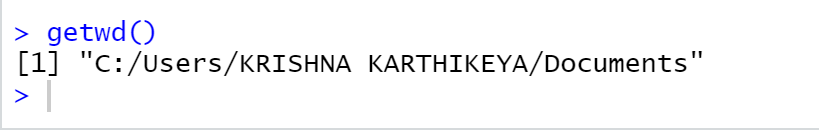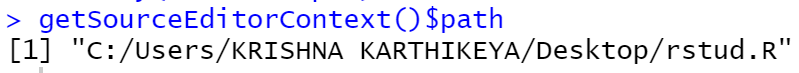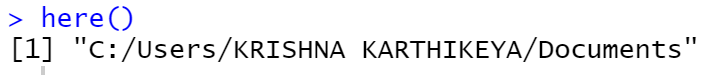How to get the path of current script in R ?

• Last Updated : 30 May, 2021

In this article, we will see how to determine the path of the current script in R Programming Language.

If we want to check the current directory of the R script, we can use getwd( ) function. For getwd( ), no need to pass any parameters. If we run this function we will get the current working directory or current path of the R script. To change the current working directory we need to use a function called setwd( ). We need to pass the path as a parameter.

syntax : getwd( )

Example :

R

 getwd()

Output :Method 2: Using rstudioapi package

To use the functions of rstudioapi we need to install this package first. To install this package type the below command in the terminal.

install.packages(rstudioapi)

From rstudioapi package we need to use getSourceEditorConext(). It’s like a list. We need to retrieve the path from it. So we need to use \$ operator along with getSourceEditorConext(). Before executing this we need to save the R script with some name and with .R extension. Then run the below code for getting the current path of R script.

Example :

R

 # importing rstudioapi packagelibrary("rstudioapi")   # retrieving path from getSourceEditorContext() # using \$ operator getSourceEditorContext()\$path

Output :Method 3: Using here Library

In the here library we are going to use here( ) function. This function determines the path of the current R script. No need to pass any parameters. Just import the library using the library( ) function. If the package is not available, install using install.packages( ) function by passing package name as parameter within quotations. After installing package, call here( ) function.

Syntax : here( )

Example :

R

 library("here")  here()

Output :My Personal Notes arrow_drop_up